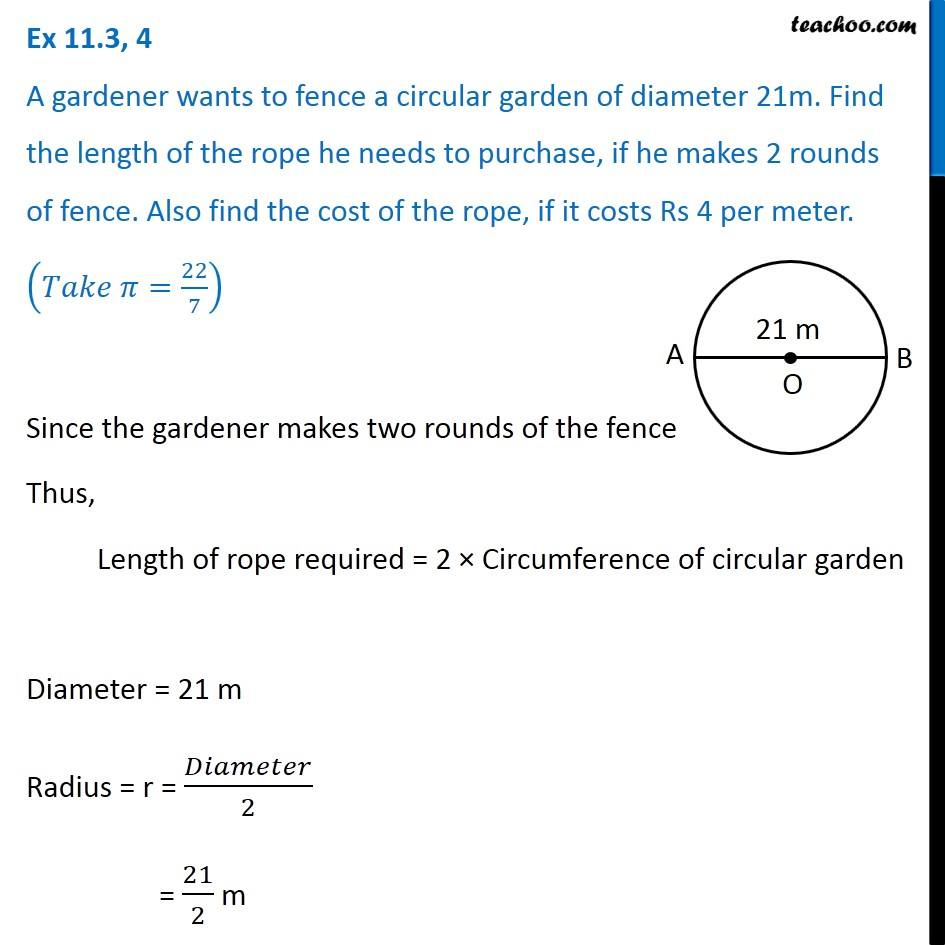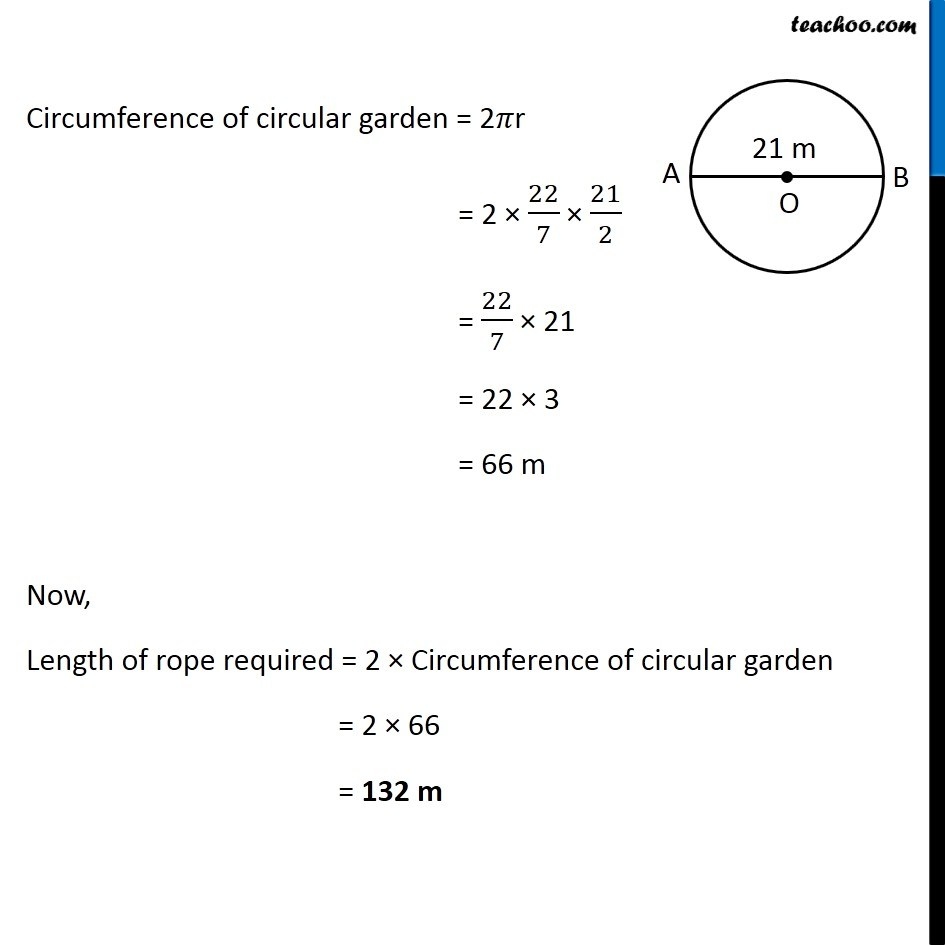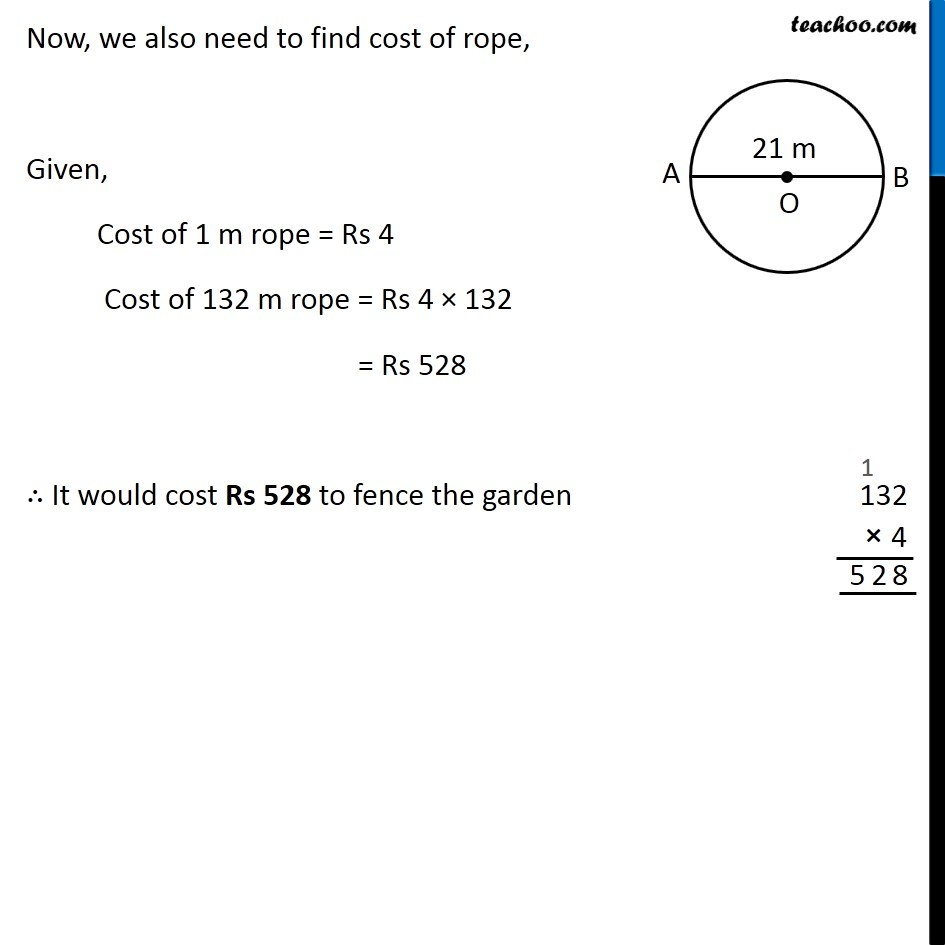1. Chapter 11 Class 7 Perimeter and Area
2. Concept wise
3. Circumference of circle

Transcript

Ex 11.3, 4 A gardener wants to fence a circular garden of diameter 21m. Find the length of the rope he needs to purchase, if he makes 2 rounds of fence. Also find the cost of the rope, if it costs Rs 4 per meter. (𝑇𝑎𝑘𝑒 𝜋=22/7) Since the gardener makes two rounds of the fence Thus, Length of rope required = 2 × Circumference of circular garden Diameter = 21 m Radius = r = 𝐷𝑖𝑎𝑚𝑒𝑡𝑒𝑟/2 = 21/2 m Circumference of circular garden = 2𝜋r = 2 × 22/7 × 21/2 = 22/7 × 21 = 22 × 3 = 66 m Now, Length of rope required = 2 × Circumference of circular garden = 2 × 66 = 132 m Now, we also need to find cost of rope, Given, Cost of 1 m rope = Rs 4 Cost of 132 m rope = Rs 4 × 132 = Rs 528 ∴ It would cost Rs 528 to fence the garden